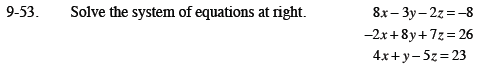Home > CCA2 > Chapter 9 > Lesson 9.2.1 > Problem9-53

9-53.
1. Solve the system of equations below. Homework Help ✎

2. 8x − 3y − 2z = −8
−2x + 8y + 7z = 26
4x + y − 5z = 23Multiply the 2nd equation by 4 and add to the 1st.

29y + 26z = 96 (Eq. #4)

Multiply the 2nd equation by 2 and add to the 3rd.

17y + 9z = 75 (Eq. #5)

Multiply the 5th equation by 26.

442y + 234z = 1950

Multiply the 4th equation by −9.

−261y − 234z = −864

Subtract the two equations eliminating y. Solve for y.

181y = 1086

y = 6

Use substitution to find x and y. Check your answers in all three equations!